## Convert the scientific notation to a decimal number. Type the correct answer in the box. 4 × 10-5 cm = cm

Question

Convert the scientific notation to a decimal number. Type the correct answer in the box. 4 × 10-5 cm = cm

in progress 0
6 months 2021-07-18T23:02:51+00:00 1 Answers 11 views 0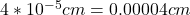Explanation:

Given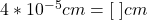Required

Solve

Apply law of indices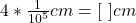Evaluate 10^5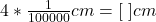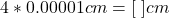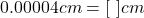Hence: